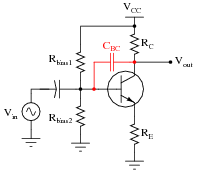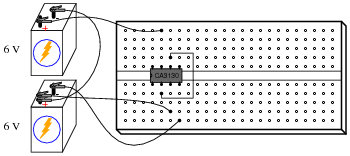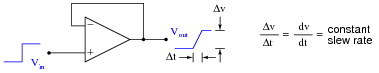# AC Negative Feedback OpAmp Circuits

• #### Question 1

 Don’t just sit there! Build something!!

Learning to mathematically analyze circuits requires much study and practice. Typically, students practice by working through lots of sample problems and checking their answers against those provided by the textbook or the instructor. While this is good, there is a much better way.

You will learn much more by actually building and analyzing real circuits, letting your test equipment provide the “answers” instead of a book or another person. For successful circuit-building exercises, follow these steps:

1. Carefully measure and record all component values prior to circuit construction.
2. Draw the schematic diagram of the circuit to be analyzed.
3. Carefully build this circuit on a breadboard or other convenient medium.
4. Check the accuracy of the circuit’s construction, following each wire to each connection point, and verifying these elements one-by-one on the diagram.
5. Mathematically analyze the circuit, solving for all voltage and current values.
6. Carefully measure all voltages and currents, to verify the accuracy of your analysis.
7. If there are any substantial errors (greater than a few percent), carefully check your circuit’s construction against the diagram, then carefully re-calculate the values and re-measure.

Avoid using the model 741 op-amp, unless you want to challenge your circuit design skills. There are more versatile op-amp models commonly available for the beginner. I recommend the LM324 for DC and low-frequency AC circuits, and the TL082 for AC projects involving audio or higher frequencies.

As usual, avoid very high and very low resistor values, to avoid measurement errors caused by meter “loading”. I recommend resistor values between 1 kΩ and 100 kΩ.

One way you can save time and reduce the possibility of error is to begin with a very simple circuit and incrementally add components to increase its complexity after each analysis, rather than building a whole new circuit for each practice problem. Another time-saving technique is to re-use the same components in a variety of different circuit configurations. This way, you won’t have to measure any component’s value more than once.

• #### Question 2

In a common-emitter transistor amplifier circuit, the presence of capacitance between the collector and base terminals - whether intrinsic to the transistor or externally connected - has the effect of turning the amplifier circuit into a low-pass filter, with voltage gain being inversely proportional to frequency:Explain why this is. Why, exactly, does a capacitance placed in this location affect voltage gain? Hint: it has something to do with negative feedback!

• #### Question 3

Which of the following amplifier circuits will be most affected by the base-collector capacitance (shown here as an externally-connected 10 pF capacitor) as frequency increases? Explain why.• #### Question 4

A common problem encountered in the development of transistor amplifier circuits is unwanted oscillation resulting from parasitic capacitance and inductance forming a positive feedback loop from output to input. Often, these parasitic parameters are quite small (nanohenrys and picofarads), resulting in very high oscillation frequencies.

Another parasitic effect in transistor amplifier circuits is Miller-effect capacitance between the transistor terminals. For common-emitter circuits, the base-collector capacitance (CBC) is especially troublesome because it introduces a feedback path for AC signals to travel directly from the output (collector terminal) to the input (base terminal).

Does this parasitic base-to-collector capacitance encourage or discourage high-frequency oscillations in a common-emitter amplifier circuit? Explain your answer.

• #### Question 5

A student connects a model CA3130 operational amplifier as a voltage follower (or voltage buffer), which is the simplest type of negative feedback op-amp circuit possible:With the non-inverting input connected to ground (the midpoint in the split 6/-6 volt power supply), the student expects to measure 0 volts DC at the output of the op-amp. This is what the DC voltmeter registers, but when set to AC, it registers substantial AC voltage!

Now this is strange. How can a simple voltage buffer output alternating current when its input is grounded and the power supply is pure DC? Perplexed, the student asks the instructor for help. Öh,” the instructor says, “you need a compensation capacitor between pins 1 and 8.” What does the instructor mean by this cryptic suggestion?

• #### Question 6

Some operational amplifiers come equipped with compensation capacitors built inside. The classic 741 design is one such opamp:Find the compensation capacitor in this schematic diagram, and identify how it provides frequency-dependent negative feedback within the opamp to reduce gain at high frequencies.

• #### Question 7

Some operational amplifiers are internally compensated, while others are externally compensated. Explain the difference between the two. Hint: examples of each include the classic LM741 and LM101 operational amplifiers. Research their respective datasheets to see what you find on compensation!

• #### Question 8

Define “Gain-Bandwidth Product” (GBW) as the term applies to operational amplifiers.

• #### Question 9

Define “Unity-Gain Bandwidth” (B1) as the term applies to operational amplifiers.

• #### Question 10

Explain the effect that compensation capacitance has on an operational amplifier’s gain-bandwidth product (GBW). Does a larger compensation capacitance yield a greater GBW or a lesser GBW, and why?

• #### Question 11

An important AC performance parameter for operational amplifiers is slew rate. Explain what “slew rate” is, and what causes it to be less than optimal for an opamp.

• #### Question 12

It strikes some students as odd that opamps would have a constant slew rate. That is, when subjected to a step-change input voltage, an opamp’s output voltage would quickly ramp linearly over time, rather than ramp in some other way (such as the inverse exponential curve seen in RC and RL pulse circuits):Yet, this effect has a definite cause, and it is found in the design of the opamp’s internal circuitry: the voltage multiplication stages within operational amplifier circuits often use active loading for increased voltage gain. An example of active loading may be seen in the following schematic diagram for the classic 741 opamp, where transistor Q9 acts as an active load for transistor Q10, and where transistor Q13 provides an active load for transistor Q17:Explain how active loading creates the constant slew rate exhibited by operational amplifier circuits such as the 741. What factors account for the linear ramping of voltage over time?

• #### Question 13

Calculate the impedance (in complex number form) “seen” by the AC signal source as it drives the passive integrator circuit on the left, and the active integrator circuit on the right. In both cases, assume that nothing is connected to the Vout terminal:• #### Question 14

Calculate the phase angle of the current drawn from the AC signal source as it drives the passive integrator circuit on the left, and the active integrator circuit on the right. In both cases, assume that nothing is connected to the Vout terminal: